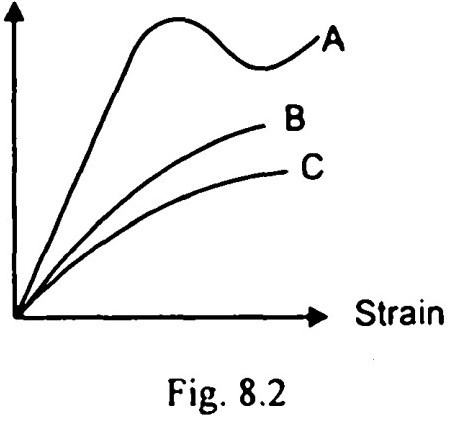Search

# Soil Mechanics (161-180)

Updated: Apr 27, 2020

161. Lime stabilisation is very effective in treating plastic clayey soils

162. Undisturbed samples are obtained by thin-walled samplers

163 Stationary piston sampler and rotary sampler are both thin-walled samplers.

164. The curves A, B and C shown in Fig. 8.2 are respectively the stress-strain curves for Stressdense sand, loose sand and clay

165. Greater skin friction retards the sinking of well

166. The bearing capacity of a strip footing on a saturated clay is 120 kN/m². The bearing capacity of a circular footing (diameter = width) will be.

more than 120 kN/m²

167. A plate load test is useful to estimate both bearing capacity and settlement

of foundation

168. The radius of friction circle or Φ-circle in friction circlp method is r sinΦ

169. The largest value of stability number is 0.261

170. A shallow foundation is defined as a foundation which has a depth of embedment less than its width

171. For an anisotropic soil, permeabilities in x and y directions are Kₓ and Ky

respectively in a two dimensional flow. The effective permeability Keq, for the soil

172. For sand of uniform spherical particles, the ratio of void ratios in the loosest and the densest states is: 2.6

173. The description of 'sandy silty clay signifies that the soil contains unequal proportions of the three constituents such that clay > silt > sand

174. Increasing the depth of foundation in saturated clays results in an increased

ultimate bearing capacity for strip footings the term in the bearing capacity

equation qNq, increases with depth.

175. A soil having particles of nearly the same size is known as: uniformly graded

176. The unit weight of a soil at zero air voids depends on:

a)specific gravity

b) water content

c) unit weight of water

177. The soils most susceptible to liquefaction are saturated fine and medium sands of uniform particle size

178. The value of bearing capacity factor for cohesion N𝒸, for piles as per Meyerhof is taken as 9.0

179. The slope of the e-log p curve for a soil mass gives compression index, C𝒸

180. If r = frequency ratio, D = damping ratio, then magnification factor in a damped forced vibration is equal to